# Inversion of an elliptic integral

(diff) ← Older revision | Latest revision (diff) | Newer revision → (diff)

The problem of constructing the functionas a function ofor of constructing single-valued composite functions of the form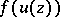in the case of an elliptic integralwhereis a rational function of variablesandwhich are related by the equation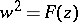, where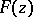is a polynomial of degree 3 or 4 without multiple roots. The complete solution of this problem was given almost simultaneously in 1827–1829 by N.H. Abel and C.G.J. Jacobi, who showed that its solution led to new transcendental elliptic functions (cf. Elliptic function).

An essentially different approach to the theory of elliptic functions is due to K. Weierstrass. For the elliptic integral of the first kind in Weierstrass normal form,turns out to be the Weierstrass-function with invariants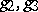(see Weierstrass elliptic functions). For the elliptic integral of the first kind in Legendre normal form,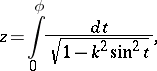inversion leads to the Jacobi elliptic functions.

How to Cite This Entry:
Inversion of an elliptic integral. Encyclopedia of Mathematics. URL: http://encyclopediaofmath.org/index.php?title=Inversion_of_an_elliptic_integral&oldid=19005
This article was adapted from an original article by E.D. Solomentsev (originator), which appeared in Encyclopedia of Mathematics - ISBN 1402006098. See original article